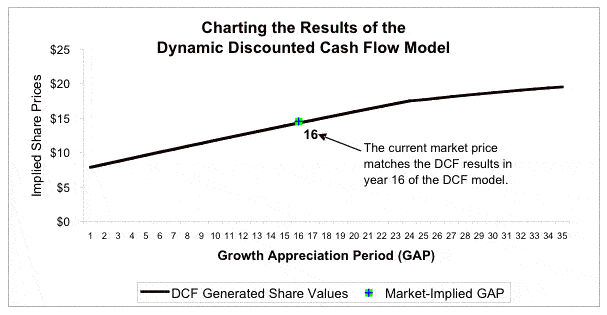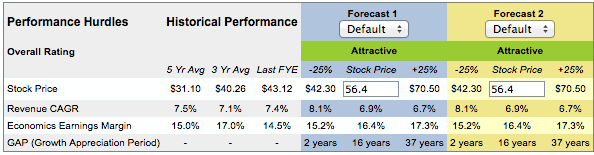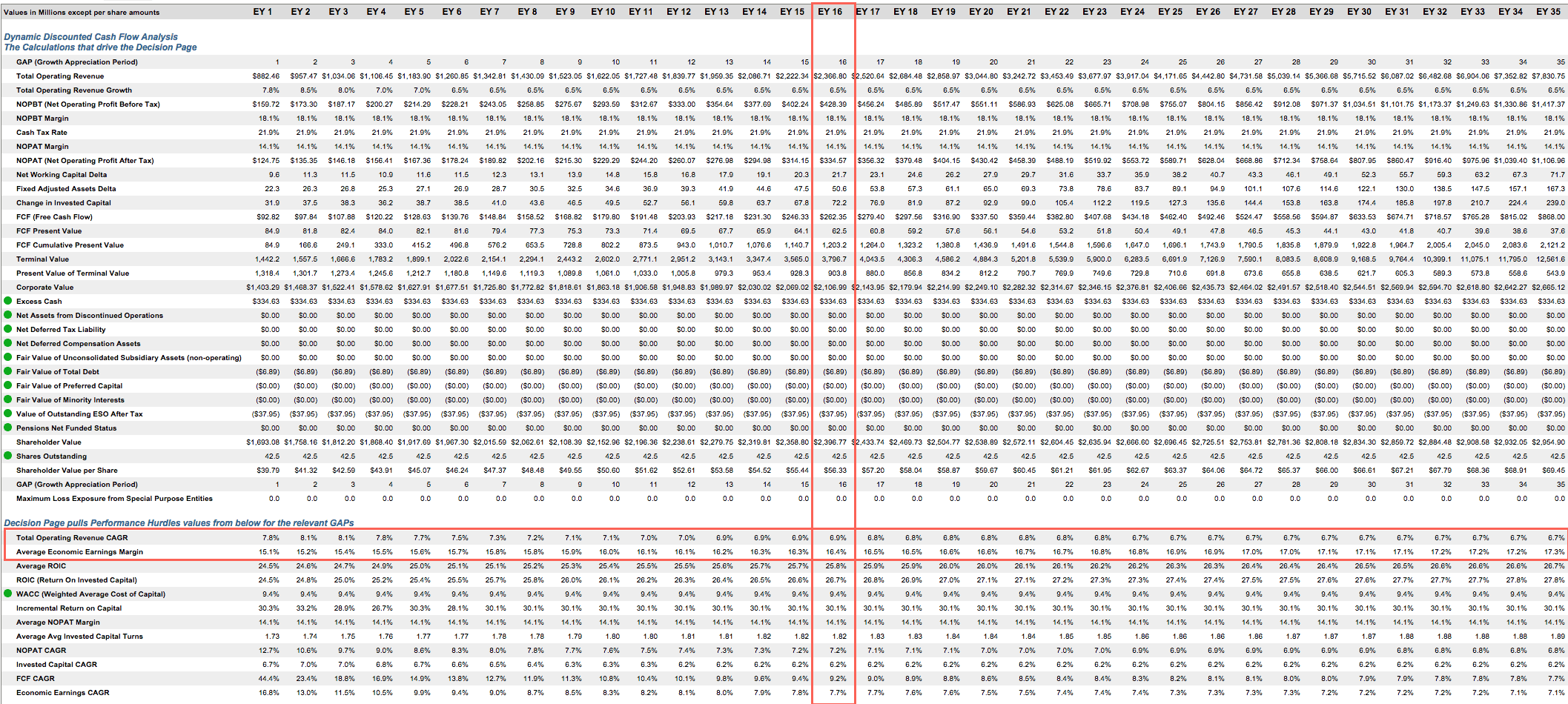## Growth Appreciation Period (GAP)

Metrics are only as good as the data that drive them. The best fundamental data in the world drives our metrics. Here’s proof from some of the most respected public & private institutions in the world.

The Growth Appreciation Period is the number of years that a business is expected to earn a ROIC greater than WACC on new investments.

GAP is the amount of time a business can grow its economic earnings. After the GAP, it is assumed that incremental investments by the business earn ROIC equal to WACC or the net present value of all investments equal zero. Warren Buffett refers to GAP as the moat around a business' castle. It is also known as the CAP (Competitive Advantage Period) and the forecast growth horizon.

Our dynamic DCF model calculates share prices attributable to multiple GAP scenarios. For example, the value of the company with a twenty-year forecast growth horizon assumes the company will enjoy a twenty-year GAP. Without a model that encompasses this long-term approach, investors are unable to assess the complete expectations embedded in any asset price.

Market-Implied GAP

The market-implied GAP is the number of years that a company's stock price implies it will earn ROIC greater than WACC on incremental investments. Provided that the estimates entered on the forecast page are based on market consensus projections, the market-implied GAP represents the forecast horizon needed in a DCF model to arrive at a value equal to the current market price.

How GAP Is Used in our Discounted Cash Flow (DCF) Model

The key difference between our DCF and others is that we calculate the value attributable to equity shareholders over multiple time periods, not just 5 or 10 years. In addition, our yearly calculations represent different GAPs because they are based on a Terminal Value that assumes the company generates no future incremental profits. To be specific, our Terminal Value for each annual calculation is a perpetuity calculation that assumes no future growth after each GAP. The formula is NOPATt+1 / WACC. Using a Terminal Value that assumes no future profit growth enables our DCF model to calculate the specific value of companies implied by each Growth Appreciation Period.

See Figure 1 for a graphic representation of how our model's dynamic discounted cash flow analysis calculates the value of a business and the attendant value available to shareholders for multiple Growth Appreciation Periods.

Figure 1: How We Use a DCF Model to Determine GAPThis chart shows how the value of the company analyzed in this example rises as its Growth Appreciation Period increases. The 'Market Implied GAP' equals the Growth Appreciation Period implied by the current market price. Our model calculates the 'Market Implied GAP' by matching the current stock price with the year that the DCF value matches that of the current stock price. For example, the 'Market Implied GAP' for the company in Figure 1 is 16 years. Our model can also calculate the GAP implied for target prices as well as any other stock prices no matter how great or small they may be. The analysis in Figure 1 shows DCF values for only 35 years though the model values companies for 100 years into the future. Figure 2 shows the DCF  info summarized on the Company Model Decision Page.

#### Figure 2: The Valuation MatrixFigure 3, below, presents a copy of the DCF model used to generate the values in Figure 2. Figure 3 also shows how our DCF model calculates values for multiple forecast horizons or GAPs. These values provide the data needed to generate a chart like the one in Figure 1 above and like the valuation matrix as presented on the Company Model Decision Page. Note the highlighted sections in Figure 3 and how they match exactly with the Revenue CAGRs, Economic Earnings Margin, and GAPs presented in Figure 2.

#### Figure 3: Sample Dynamic DCF